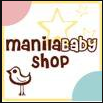### Next9 Resellers

You can get your favorite Next9 products from the following resellers!!

Mama.Baby.Love sells nursing wear plus lots more! They carry our slings, nursing covers and cloth diapers.

=o=o=o=o=o=o=o=o=o=o=o=o=o=o=o=o=o=o=o=o=o=o=o=o=o=o=o=o=o=o=o=o=o=o=

Cherub's Nest

Charm Ang
0918 337 1169

=o=o=o=o=o=o=o=o=o=o=o=o=o=o=o=o=o=o=o=o=o=o=o=o=o=o=o=o=o=o=o=o=o=o=

Caleb's Closet Ecostore
 Caleb's Closet Ecostore is a one-stop online shop offering modern solutions to natural parenting.  Run by a discriminating doctor mom, she put it up to carry the stuff she loves and believes in.  Totally into breastfeeding, attachment parenting, and ecological sound options.
0922 862 5521
=o=o=o=o=o=o=o=o=o=o=o=o=o=o=o=o=o=o=o=o=o=o=o=o=o=o=o=o=o=o=o=o=o=o=

Ethan's Store

Joey Ver
ethansstore@gmail.com
0905-8654101

=o=o=o=o=o=o=o=o=o=o=o=o=o=o=o=o=o=o=o=o=o=o=o=o=o=o=o=o=o=o=o=o=o=o=

Indigo Baby

INDIGObaby is a collaboration of life saving products to enrich you and your baby's life.
=o=o=o=o=o=o=o=o=o=o=o=o=o=o=o=o=o=o=o=o=o=o=o=o=o=o=o=o=o=o=o=o=o=o=

Earthlings Mama

The Mothering Earthlings online shop is a haven of fashion statements for kids.  Here, young earthlings make the style rules.

They carry our slings, nursing covers and cloth diapers.

=o=o=o=o=o=o=o=o=o=o=o=o=o=o=o=o=o=o=o=o=o=o=o=o=o=o=o=o=o=o=o=o=o=o=

Pinoy Baby Store

Rowie Palacios is a cloth diapering mama, check out Next9 Cloth Diapers in her store!

=o=o=o=o=o=o=o=o=o=o=o=o=o=o=o=o=o=o=o=o=o=o=o=o=o=o=o=o=o=o=o=o=o=o=

Mommy and Baby Essentials

Theresa Orteza
0928 5004552

=o=o=o=o=o=o=o=o=o=o=o=o=o=o=o=o=o=o=o=o=o=o=o=o=o=o=o=o=o=o=o=o=o=o=Manila Baby Shop

Check out Next9 Products alongside their famous diaper bags!!

manilababyshop@gmail.com
0917.533.4209

=o=o=o=o=o=o=o=o=o=o=o=o=o=o=o=o=o=o=o=o=o=o=o=o=o=o=o=o=o=o=o=o=o=o=

Wonder World Inc. - Acropolis Clubhouse and Jump Street in Robinson's Galleria!
They carry our slings and cloth diapers.

=o=o=o=o=o=o=o=o=o=o=o=o=o=o=o=o=o=o=o=o=o=o=o=o=o=o=o=o=o=o=o=o=o=o=

Babyland
Branches: Facilities Center, Shaw Boulevard, Glorietta 2, Festival Mall, Eastwood Mall
They carry our slings, nursing covers and cloth diapers.

=o=o=o=o=o=o=o=o=o=o=o=o=o=o=o=o=o=o=o=o=o=o=o=o=o=o=o=o=o=o=o=o=o=o=

Rustan's
Branches: Makati, Shang Tower, Gateway, Alabang and Cebu
They carry our slings and nursing covers.

=o=o=o=o=o=o=o=o=o=o=o=o=o=o=o=o=o=o=o=o=o=o=o=o=o=o=o=o=o=o=o=o=o=o=

SM Department Store and Baby Company (Podium, Rockwell and North EDSA Annex)
They carry our slings.

=o=o=o=o=o=o=o=o=o=o=o=o=o=o=o=o=o=o=o=o=o=o=o=o=o=o=o=o=o=o=o=o=o=o=